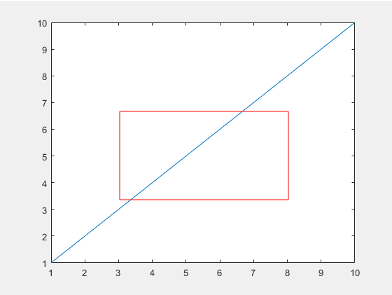# rbbox

Create rubberband box for area selection

## Syntax

```rbbox rbbox(initialRect) rbbox(initialRect,fixedPoint) rbbox(initialRect,fixedPoint,stepSize) finalRect = rbbox(...) ```

## Description

`rbbox ` initializes and tracks a rubberband box in the current figure. It sets the initial box as a 0-by-0 pixel rectangle, anchors the box at the figure's `CurrentPoint`, and begins tracking from this point.

`rbbox(initialRect) ` specifies the initial location and size of the rubberband box as `[x y width height]`, where `x` and `y` define the lower left corner, and `width` and `height` define the size. `initialRect` is in the units specified by the current figure's `Units` property, and measured from the lower left corner of the figure window. The corner of the box closest to the pointer position follows the pointer until `rbbox` receives a button-up event.

`rbbox(initialRect,fixedPoint) ` specifies the corner of the box that remains fixed. All arguments are in the units specified by the current figure's `Units` property, and measured from the lower left corner of the figure window. `fixedPoint` is a two-element vector, ```[x y]```. The tracking point is the corner diagonally opposite the anchored corner defined by `fixedPoint`.

`rbbox(initialRect,fixedPoint,stepSize) ` specifies how frequently the rubberband box is updated. When the tracking point exceeds `stepSize` figure units, `rbbox` redraws the rubberband box. The default stepsize is `1`.

`finalRect = rbbox(...) ` returns a four-element vector,` [x` `y` `width` `height]`, where `x` and `y` are the x and y components of the lower left corner of the box, and `width` and `height` are the size of the box.

## Examples

collapse all

To interactively create an annotation rectangle in a figure, first create a program file called `createRectangle.m`. Within the program file:

• Create a figure. Normalize the units of the figure by setting its `Units` property.

• Block statements from executing until you click a mouse button using the `waitforbuttonpress` function.

• Initialize a rubberband box using the `rbbox` function. Make a rectangle by clicking and dragging. When you release the mouse button, the `rbbox` function returns the position of the rectangle.

• Display the rectangle using the `annotation` function.

```function createRectangle figure('Units','normalized') waitforbuttonpress pos = rbbox; annotation('rectangle',pos,'Color','r') end```

Run the program file. Create the rectangle by clicking and dragging.

`createRectangle`To create an annotation rectangle within an `Axes` object, you must first disable built-in interactions. Otherwise, when you drag the rectangle, the axes will pan (in a 2-D view) or rotate (in a 3-D view). For more information about built-in interactions, see Control Chart Interactivity.

Create a program file called `createRectangleInAxes.m`. Within the program file:

• Return the current figure as a variable and normalize its units.

• Block statements from executing until you click a mouse button using the `waitforbuttonpress` function.

• Initialize a rubberband box using the `rbbox` function. Make a rectangle by clicking and dragging. When you release the mouse button, the `rbbox` function returns the position of the rectangle.

• Display the rectangle using the `annotation` function.

```function createRectangleInAxes f = gcf; f.Units = 'normalized'; waitforbuttonpress pos = rbbox; annotation('rectangle',pos,'Color','r') end```

Then, create a chart. Disable built-in interactions by calling the `disableDefaultInteractivity` function.

```plot(1:10) ax = gca; disableDefaultInteractivity(ax) ```

Call the program file and create the rectangle.

```createRectangleInAxes ```After creating the rectangle, you can reenable built-in interactions by calling the `enableDefaultInteractivity` function.

`enableDefaultInteractivity(ax)`

## Tips

`rbbox` is useful for defining and resizing a rectangular region:

• For box definition, `initialRect` is `[x y 0 0]`, where `(x,y)` is the figure's `CurrentPoint`.

• For box resizing, `initialRect` defines the rectangular region that you resize (e.g., a legend). `fixedPoint` is the corner diagonally opposite the tracking point.

`rbbox` returns immediately if a button is not currently pressed. Therefore, you use `rbbox` with `waitforbuttonpress` so that the mouse button is down when `rbbox` is called. `rbbox` returns when you release the mouse button.

## Version History

Introduced before R2006a

expand all

Behavior changed in R2020b